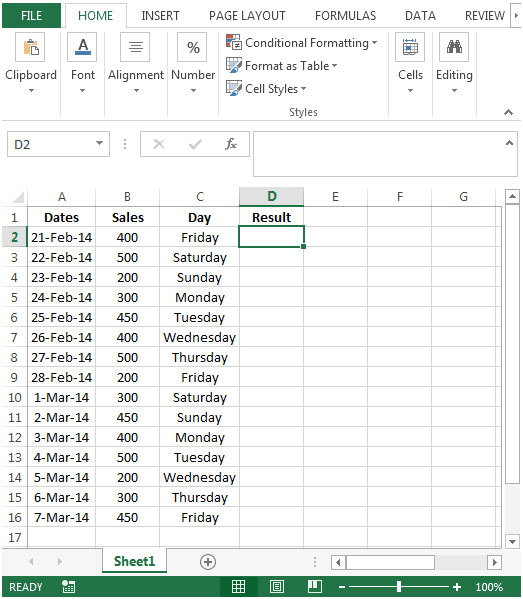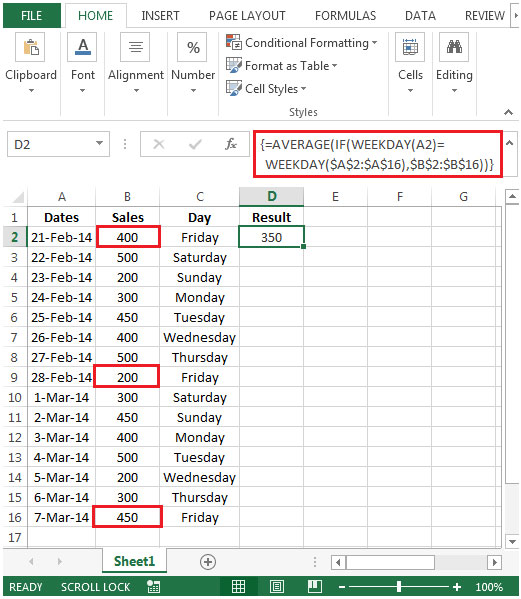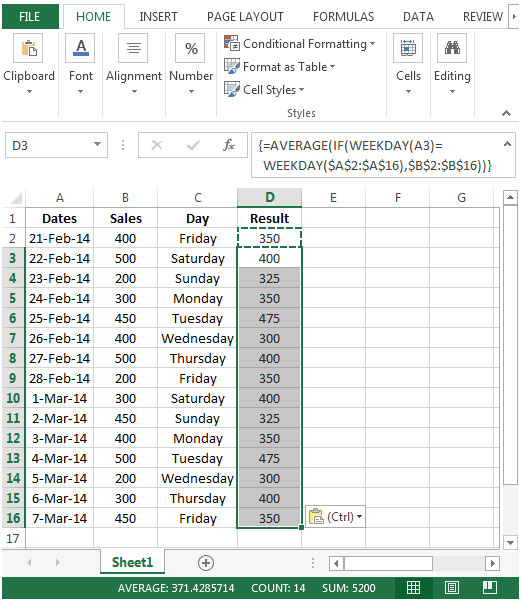Averaging Sales Totals By Day Of The Week In Microsoft Excel 2010

In this article, we will learn how to find average sales totals by day of the week in Microsoft Excel 2010.

To find the average sales total by particular day of the week, i.e. we want to determine which day of the week corresponds with each date in column A, and then calculate an average sales figure for each day of the week over the whole period. That is; we want an average sales total for all of the Mondays, one for all the Tuesdays, and so on.

We will use a combination of AVERAGE, IF & WEEKDAY functions to extract the output.

AVERAGE function can be used to find the average value or arithmetic mean of values in a selected range of cells.

Syntax: =AVERAGE(number1,number2,…)

Arguments

number1, number2,……number n are numeric values. They can be numbers or names, arrays, or references that contain numbers.

IF function checks whether the condition you specify is TRUE or FALSE. If the condition returns TRUE then it returns preset value, and if the condition returns FALSE then it returns another preset value.

Syntax = IF(logical_test,value_if_true,value_if_false)

logical_test: Logical test will test the condition or criteria. If the condition meets then it returns the preset value, and if the condition does not meet then it returns another preset value.

value_if_true: The value that you want to be returned if this argument returns TRUE.

value_if_false: The value that you want to be returned if this argument returns FALSE

WEEKDAY: It returns a number from 1 to 7 identifying the day of the week of a date

Syntax: =WEEKDAY(serial_number,return_type)

serial_number: This represents the date of the day that you want to find.

return_type: This is optional. A number that determines the type of return value.

Let us take an example:

• We have some dates in column A & sales on these days in column B.
• We have used TEXT function in column C to look for the day (Monday to Sunday). In cell C2, enter the function as =TEXT(A2,”dddd”)& copy down in below range C3:C16.
• We need a formula in column D to find the average sales total by any specific day of the week.• In cell D2, the formula would be

{=AVERAGE(IF(WEEKDAY(A2)=WEEKDAY(\$A\$2:\$A\$16),\$B\$2:\$B\$16))}

This is an array formula, which requires formula to be enclosed with curly brackets by using CTRL + SHIFT + ENTER.• We get the average sales for Friday 350 as =AVERAGE(400,200,450)
• Copy down the formula from cell D2 to range D3:D16. While copying, you need to select cell D2 first, and then you need to select range D3:D16 & then paste the formula, and you will get the desired result.## Users are saying about us...

1.Bill McNicoll

Why does the data in column B not appear in the formula? Also, I am running Excel 2007 and the WEEKDAY function has two required arguments.

Terms and Conditions of use

The applications/code on this site are distributed as is and without warranties or liability. In no event shall the owner of the copyrights, or the authors of the applications/code be liable for any loss of profit, any problems or any damage resulting from the use or evaluation of the applications/code.